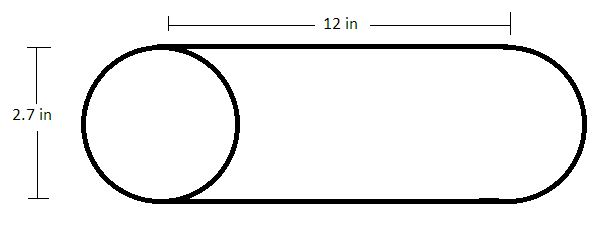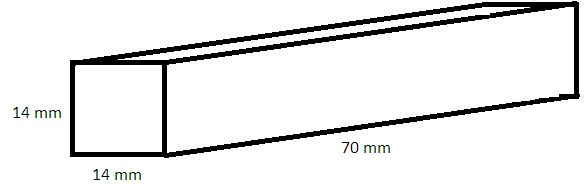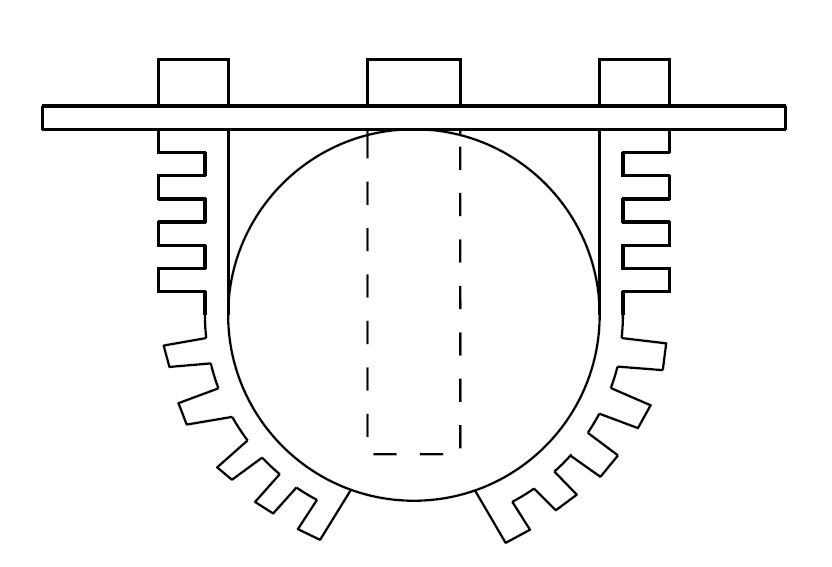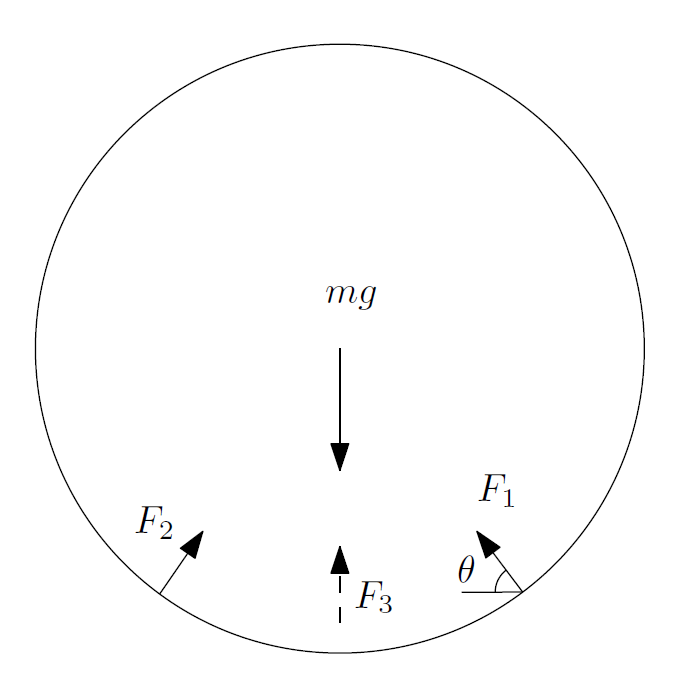## Air Tanks and Pressure:

Estimated system volumeSimplified inflatable arm schematic (fully inflated)Simplified inflatable finger schematic (fully inflated)Air storage tank capacity should be at least 2 times the estimated total volume ().

A larger tank would be preferable for longer operations, but cost and weight factors have a higher priority. As such a proposed system would be to use a smaller tank and re-circulate the air (close loop).The air compressor can be used to compress air during both inflation and deflation (robotic hand actuation). Also, the compressor can be used to compress atmospheric air into the tank to maintain a suitable tank pressure (). A 4-way solenoid valve would be used to direct the flow of air.

## Force Requirements:

Object to pick up: Tennis ball - 60 grams, 6.858cm radius

Usable finger length: 9.225 cm

Minimum “fingers”: 3Hypothetically Setup to Pick up Ball and Free Body Diagram

Assuming the first part of the finger drops straight down, the usable length of the finger will drop straight down and then wrap around the ball. The straight portion will take up the radius length of the finger length and the remaining portion can be calculated using the arc length over which the finger is in contact with the ball. This can be used to determine the angle the force is acting at through the following derivation. This assumes the force is acting normal to the surface.A force balance can then be performed to determine the minimum force needed to hold the ball. It is assumed that all fingers act on the ball equally.As seen above, the minimum force that will be needed to hold the ball in this position is 0.58 newtons. Although this exact setup may not be used, this will give a general idea about how much force is needed to grasp the ball.

## Drive System

Torque required

• Estimate of weight of the system (40lbs max)
• Size of wheels (the 4 in wheels will require less torque for the system)
• Maximum Required Torque (for the whole robot) = (Radius of Wheel) x (Max Weight of System)
• T = 2in x 40lbs (16oz/1lb) = 1280 oz/in of Torque
• Divide Max Torque by the number of drive motors:
• Torque per Motor = 1280 oz/in / 2 = 640 oz/in at the wheels.

640 oz/in at the wheels

* Both motors will require the same gearbox to increase the torque to meet the requirement, however, the SIM motor is a much simpler circuit and only requires speed control vs the stepper motor, which requires a complicated step control.

## Weight for system

 Category Weight (lbs) Component Functional 3 Compressor 1 Air Tank 3 Power System 5 Arm Structural Support Mobility 8 Frame 14 Battery 5 Motors 1 Gear Box Total 30

## Power

Current Requirements

•     Main modes of operation
• Compression stage
• Current Draw  ~ 11 amps
• Navigation stage.
• Minimum Current Draw ~ 40 amps
• Battery Drained ~ 15 minutes of continuous use.

Potential Requirements

• Microcontroller ~ 5V
• Motors            ~ 12V
• Compressor    ~ 12V

*   A 12 volt lead acid battery will most likely be used due to the high discharge rate and the relatively cheap cost of the battery. Other types of batteries such as NiMH would not have a high enough discharge rate to power the motors in the navigation stage. On the other hand, LiPo cells would be able to sustain a sufficient discharge rate but the overall cost of the battery is much higher.